# Price Appreciation

Price appreciation means an increase in the value of the investment in real estate over a period.

Appreciation describes the growth in an asset's value over time. Price increases can result from various factors, including changes in inflation or interest rates, lower supply, or increased demand.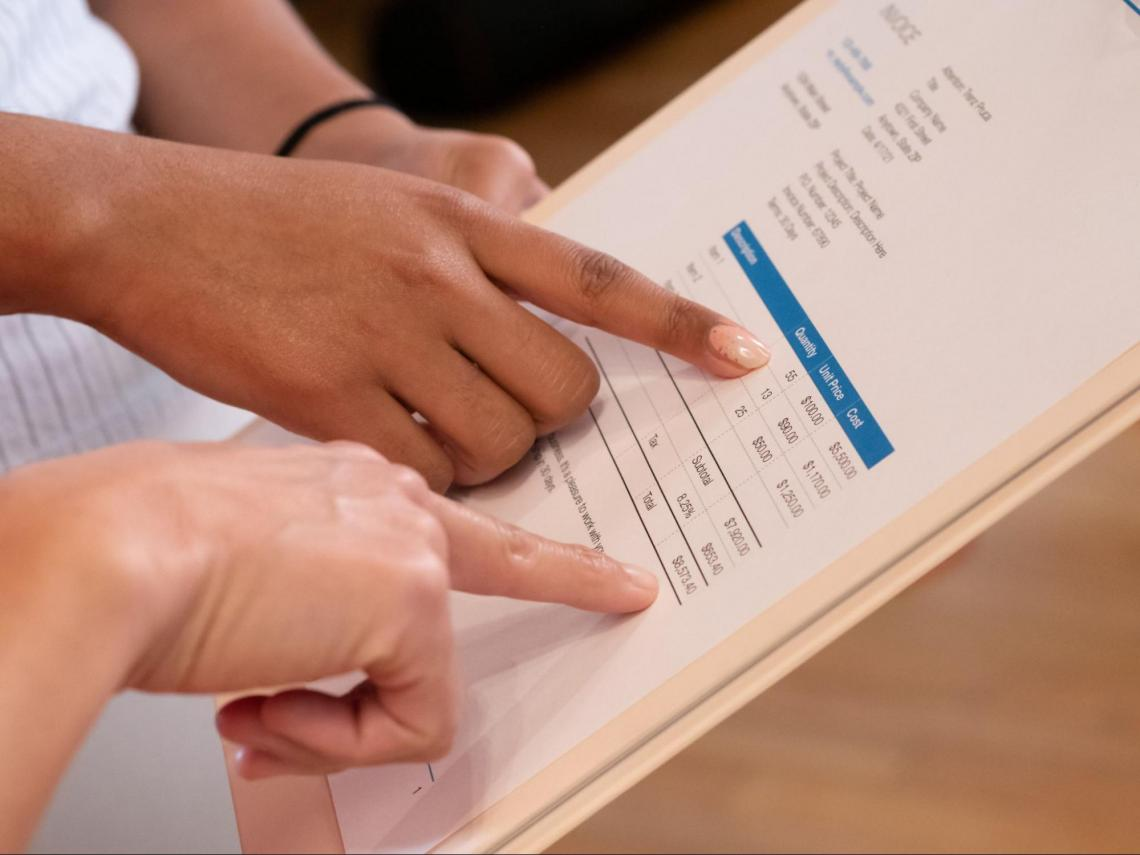Growth in any sort of assets, such as a stock, bond, currency, or real estate, is referred to as 'Price appreciation'.

For instance, the term' capital increase' describes a rise in the value of financial assets like stocks that can happen for several reasons, including an organization's improved financial performance.

The mere fact that an asset's value increases does not mean the owner is aware. When the owner revalues the item at a higher price on their financial accounts, they realize the increase.

Real estate properties' value usually increases periodically over time and is referred to as price appreciation in real estate. One of the objectives of real estate investing is to get a favorable return on investment when the investor decides to sell the property later.

A property's increase in value can be influenced by several variables, including its location, future development plans, physical structure, and the demand and supply of property in a certain area.

Property owners can also force property appreciation by doing repairs and upgrades on the property.

## Appreciation v/s Depreciation

It is beneficial to comprehend them across a wide range of businesses because they are frequently focused on when making a significant buy or investment.

For instance, by using appreciation, accountants can determine a positive adjustment to the initial value of an asset that is recorded on a company's accounting records. A negative reduction to an asset's value, on the other hand, is used as depreciation.

The price of an asset with a limited usable life typically depreciates instead of increasing in value.

When an item, such as a piece of machinery that has been utilized for a certain amount of time begins to lose economic value, depreciation is often taken into account.The assets that are bought with the hope that their value will increase over time include real estate, equities, and precious metals (Audemars Piguet, Rolex, and Patek Philippe watches).

In contrast, the worth of cars, computers, and physical equipment steadily decrease throughout their useful lifetimes.

One can stretch the expense of an item across its useful life by understanding depreciation and knowing how to calculate it. This enables businesses to estimate an asset's revenue potential accurately and calculate its cost.

One can also make adjustments to the operating earnings and cash flow in their accounting records.

The revenue (or net worth) might be impacted if one doesn't know how much their asset depreciates since one could make the mistake of buying an item that ends up costing more than the income (or value) it generates.

## Formula and Examples of Price Appreciation Calculation

Depending on the data you have at hand, there are two algorithms for determining appreciation:

• Current
• Future

You can determine the current value of an investment if you just know its original and current values.

You may get the expected future value by computing the average yearly appreciation rate and the present value.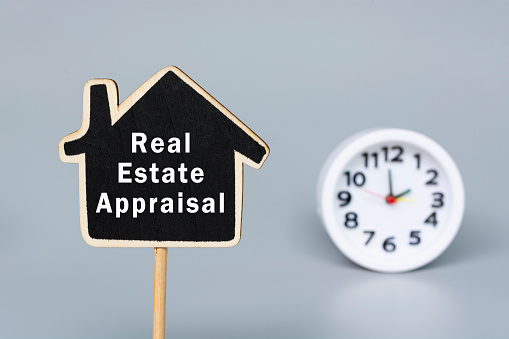The beginning and end values may be the only pieces of information you have to work with. If so, you may calculate the monetary value or percentage of appreciation using this information.

Change in value = Final value - Initial value

Appreciation Percentage = (Change in value / Initial investment) * 100

However, you might be able to leverage a ton of past public data for other investments. Investors can predict the value of future appreciation using this historical data.

Appreciation value after N years = (Appreciation factor) * (current value)

Appreciation factor = (1.0 + appreciation rate)^N number of years

Example on calculating current appreciation

Take the formula for the dollar amount and replace the formula values with the ones you are familiar with.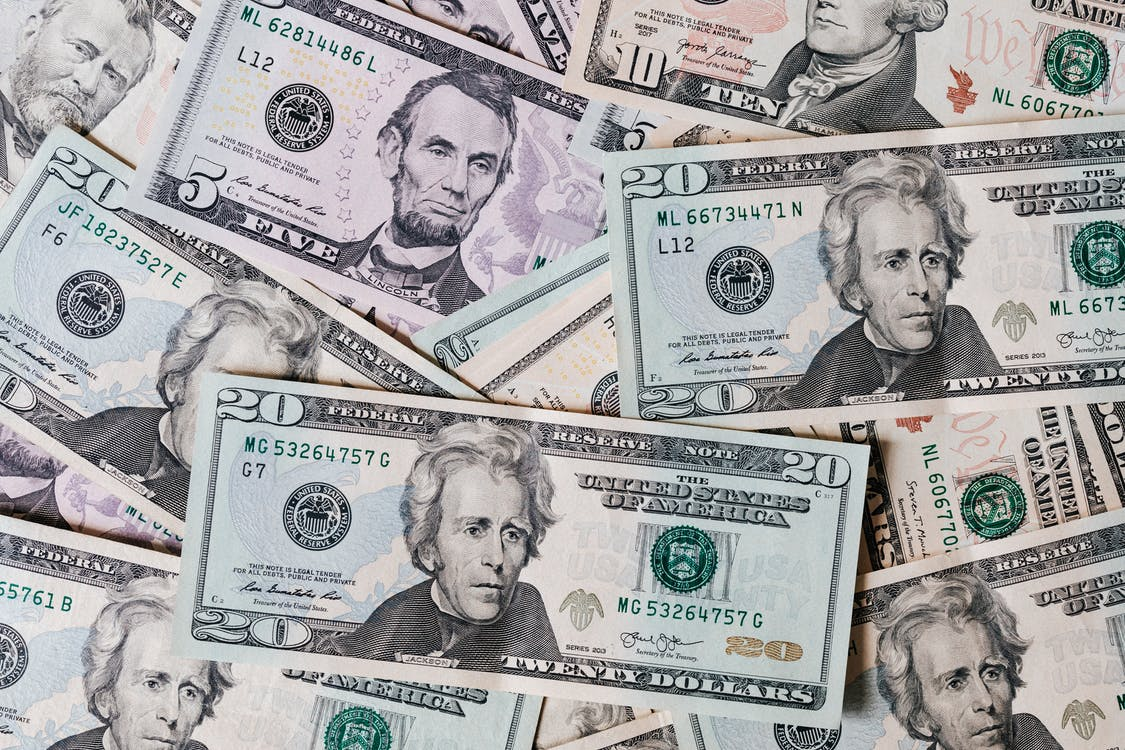For instance, the value of the apartment was \$500,000 when a real estate investor bought it, but now it is \$550,000.

Since,

Dollar based change in value = Final value - Initial value

Therefore,

Change in value = \$550,000 - \$500,000 = \$50,000

Then, to find appreciation %,

Appreciation % = (Change in value / Initial value) 100

Therefore, Appreciation % = (\$50,000 / \$500,000) 100 = 10%

The price of the apartment may be assessed by looking at the formulae that were solved above. It cost \$500,000, and now it is worth \$550,000 after a 10 percent value increase, or a \$50,000 appreciation.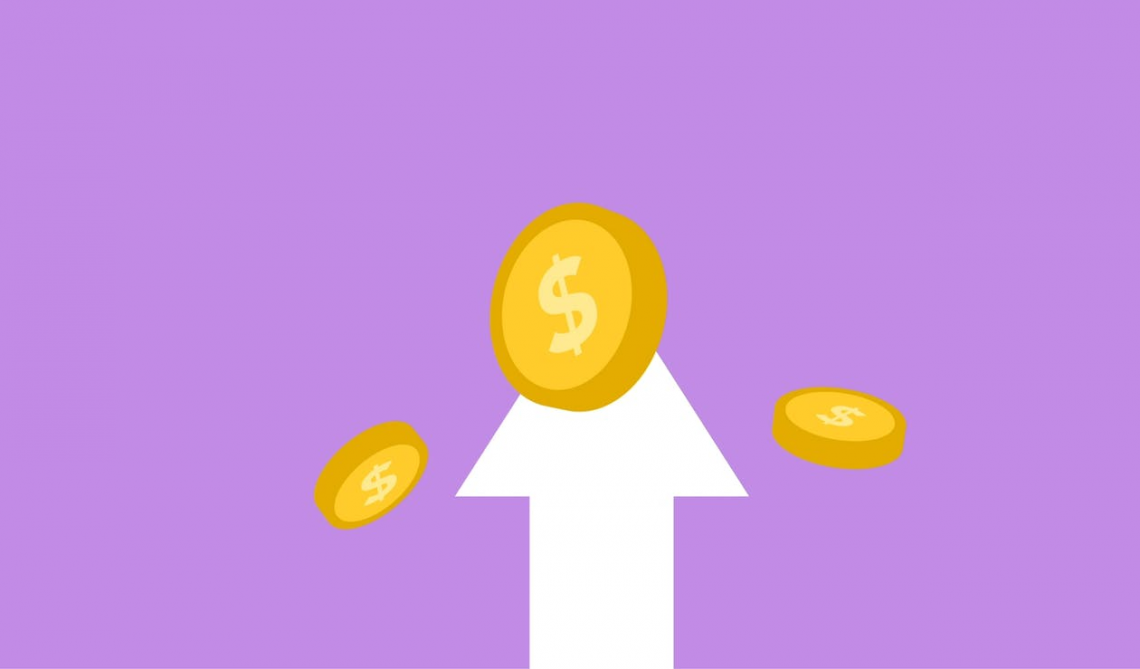Example on calculating future price appreciation

Using the aforementioned example, you now know that the apartment's price has appreciated at a rate of 10 percent.

For instance, let's consider this appreciation occurred after three years of purchase, so,

Appreciation Factor (AF) = (1.0 + appreciation rate) ^ N number of years

I.e., AF = (1.0 + 0.1) ^ 3 = (1.1) ^ 3 = 1.331

Value Appreciation (VA) after N years = (Appreciation Factor) * (current value)

Therefore, VA after N years = (1.331) * (\$550,000) = \$732,050

You may estimate the projected future appreciation of the apartment 3 years from now by looking at the formula you just computed. Your business space is currently worth \$410,000, and in five years, it will increase in value by 1.331 times, amounting to \$732,050.

It's crucial to keep in mind that this figure is only an estimate based on the supposition that the historically observed appreciation rate of 10 percent won't change.

The calculation does not consider economic shifts and other mitigating factors.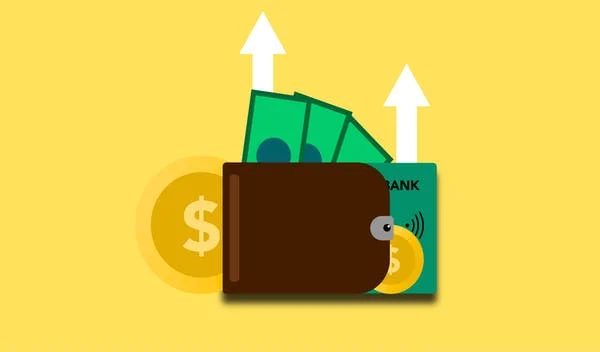## Appreciation of Currencies and Capital

1. Currency appreciation

The value of one currency rising over another is known as currency appreciation. For instance, if the EUR-USD exchange rate rises from 1.00 to 1.20, it indicates a 20% increase in the value of the euro relative to the US dollar.

Gains in domestic currency reduce exporters' earnings since their sales are worth less when translated to domestic currency.Exporters would seek to increase selling prices overseas in such circumstances to increase their profits, but they would probably experience a decline in export sales.

Additionally, a stronger currency means that foreign corporations selling will put more pressure on domestic enterprises to compete in their markets.

2. Capital Appreciation

An incentive or deferred payment arrangement, known as a stock appreciation, right connects a portion of your income to the performance of the shares of your firm.

It grants you the right to receive the cash equivalent of the increase in value of a specific number of shares over a particular period.

A stock's price rises if there is a greater demand (buyers) than supply (sellers) for it. On the other hand, if there were a bigger supply than there was a demand for a stock, the price would drop. Although forecasting the market is difficult, understanding supply and demand are simple.Consider an investor who decides to go long on TESLA stock and buys a \$100 stock of the company that pays a \$1 yearly dividend or has a 1% dividend yield.

One year later, a dividend of \$1 has been paid to the investor, and the stock is now worth \$220 per share.

The stock moved from a purchase price or cost basis of \$100 to a current market value of \$220, giving the investor a return on investment of \$120. Thus, expressed in percentage figures, an increase in stock price resulted in a return on capital of 120 percent.

And with a \$1 dividend yield,

The total return would be 120 + 1 = \$121

3. Real Estate Price Appreciation

It is described as the gradual increase in the value of a home, piece of land, or investment property. If a property's worth increases, the owner may benefit when selling it or get more money each month in rent from their renters. More equity in the property is also a result of rising home values.

Key Takeaways

1. Value increase refers to a growth in a property, capital, or currency worth over time.
2. A property's price appreciation depends on several variables, including supply and demand, interest rates, location, and potential future development.
3. Some investors buy real estate intending to eventually sell it for a profit on the increase in value while the supply is limited.
4. An increase in the value of one currency in comparison to another is known as currency appreciation. For many factors, including governmental policies, interest rates, trade balances, and business cycles, currencies appreciate against one another.
5. Over time, any asset you own will either increase in value or decrease in value. Simply said, appreciation happens when your item increases in value in any way. On the other hand, depreciation describes a loss in value.
6. When figuring out how much an investment has increased in value, start with the investment's final value and subtract its starting value to get the value change.
7. To calculate the percentage of an investment's appreciation, multiply the initial value by the value change, then multiply the result by 100.
8. For other investments, you might be able to use historical public data extensively. Using this past information, investors may forecast the worth of future appreciation.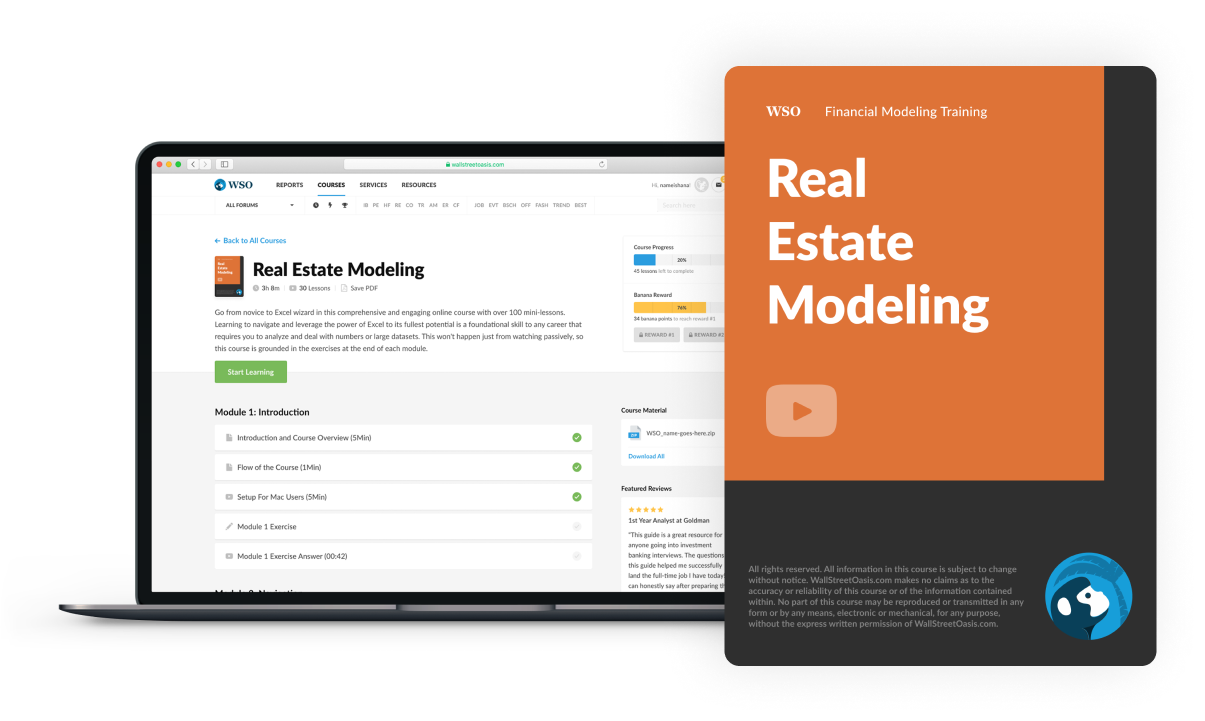### Everything You Need To Build Your RE Modeling Skills

To Help You Thrive in the Most Rigorous RE Interviews and Jobs.